# Conical Mill Grinding Tests

In the laboratory work described in the following pages, a 4 ½-ft. Hardinge mill with three removable cylindrical sections, 16 in. each in length, was used. Fig. 1 shows the mill with three cylindrical rings in place. This combination allows a mill 4 ½ ft. by 0 in., 4 ½ ft. by 16 in., 4 ½ ft. by 32 in., or 4 ½ ft. by 48 in., as desired. The conical and cylindrical sections were built of cast iron, 1 ¼ in. thick and were lined with chrome steel lifting bars 2 ½ in. high, 3 in. wide and 16 in. long, set on 11-in. centers. The head bearing was adjustable in height, thus allowing the mill to be tilted any desired amount.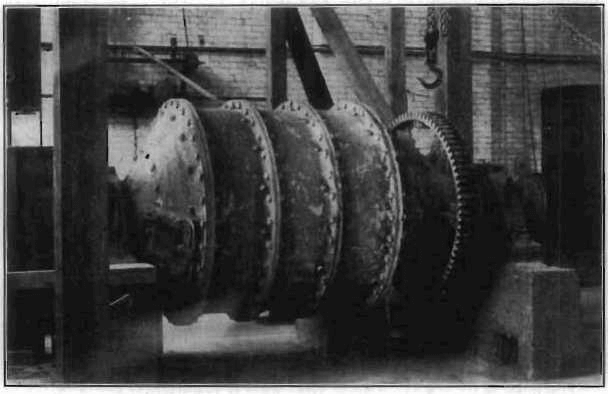Fig.1- Hardinge Mill Used in Grinding Tests

Material:

A majority of the tests were made on quartzite and trap. The quartzite contained an appreciable amount of white mica in flakes 1 to 2 mm. (0.04 to 0.08 in.) diameter, which made it rather easy to crush in the coarser sizes but difficult to grind when the finer sizes were reached. The trap was a variety of diabase quarried locally for road metal. The other materials tested (see tests 230 to 236) were of a special nature and will be described more particularly in connection with the record of the work done upon them.

Method of Feeding:

The material to be ground in any given test was weighed up and divided into lots, each lot being sufficient to furnish the feed for the mill for 5 min. In general, this lot was divided by eye into five, portions, so that a portion could be dumped into the feed box every minute. This procedure, aided by the low capacity of the scoop feeder on coarse material, assured a practically uniform feed rate. When, as was the case with finer materials, the scoop tended to take up the material meant for a 1 min. portion in two or three revolutions, the method of feeding was so changed as to take away from the scoop the burden of regulating the feed for even such a short interval as 1 min. The importance of this insistence on regular feed will be seen in Fig. 10, which presents a com­parison of the feed and discharge rates of the mill, dry crushing. In wet feeding the same methods of introducing the rock were followed. The water was introduced into the feed box from a calibrated orifice at the proper rate to give the desired moisture percentage and the result was checked by moisture samples of the discharge.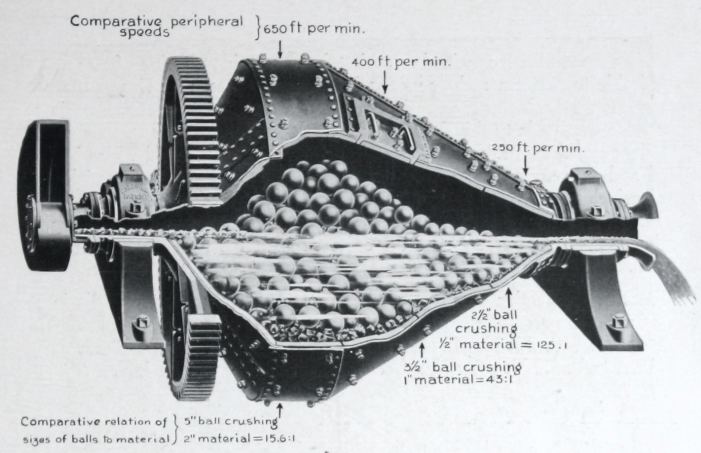Sampling:

Feed samples were taken, in every case, by the method of alternate shovels. Large samples were cut to insure accuracy. Samples of the product consisted of the whole discharge stream caught for varying inter­vals according to the feed rate. The interval for wet samples was rarely less than 1 min. For dry samples the interval was never less than 1 min. and in all cases where the feed rate was less than 1 ton per hour the sample of the product consisted of the whole discharge for an interval of 5 min.

Screen Testing:

Screen tests on feed samples were made in duplicate. The accuracy of the sampling was accepted as sufficient when cumulative graphs of the  tests were closely coincident. Product samples were passed over the 6.680-mm. (0.26-in.) sieve. The total oversize on this sieve was then run through the coarser series. The undersize of the 6.680-mm. sieve was riffled down to not less than 200 grams and then run through the balance of the Tyler Standard Sieve Scale series of screens (1.414 ratio). The amounts of the aliquot parts of the whole sample remaining on these fine sieves and passing the last (0.074 mm.) were then calculated back into terms of the whole sample and the percentages given in Table.2 were cal­culated from the figures thus determined. Duplicate samples of the riffled undersize were run occasionally in order that frequent screen tests might not breed carelessness. In no case was the difference between duplicates greater than that to be expected in grading analyses.

Moisture Determination:

Moisture determinations were made on products only, as the feed was, in every case, so dry as to be dusty. In all cases the weight of the solid plus water in the sample and the weight of the dry solid were determined by direct weighing and the percentage of moisture calculated from these figures.

Power Measurements:

Belt drive was used for the testing work. The power transmission is not so efficient, of course, as direct drive through herring-bone gears and the latter installations will give higher relative mechanical efficiencies than those recorded in this paper. The watt-hour meter and the voltmeter and ammeter were read at 5-min. intervals. The watt-hour meter readings are the basis for the figures of power consumption used, the readings of the indicating instruments being used for purposes of check only.

Outline of Testing Work Objects:

The specific object of the work described in the following pages was the determination of a set of constants and characteristic curves for the conical mill which could be applied to any installation. The 4½-ft. mill is large enough to do any class of work for which the conical mill is suited, its only limitation being a question of capacity. It was hoped to cover the question of variation in capacity due to variation in diameter by a few tests on mills of other sizes. It has, however, been impossible to do this, and the writer can offer but a tentative rule based on figures col­lected by correspondence.

Plan of Work: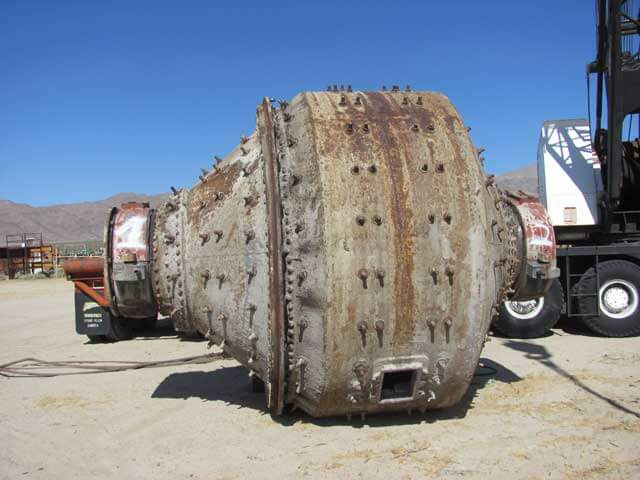The plan of the work was to start with some given set of conditions for instance, a 16-in. cylindrical section, 4,006-lb. load of mixed balls, a trap rock feed of a given size, no moisture, mill level (Test 202); and keeping these conditions constant, vary one other condition, (Tests 203 and 204 in this case the feed rate, and determine the effect of this variation on the character of the product, the horsepower, and the relative mechanical efficiency, By varying in similar manner the size of the feed, the kind of rock fed, the percentage of moisture, length of cylindrical section, slope of the mill, and the character and weight of the crushing charge, the effects of such variations on the performance of the 4½-ft. mill were determined.

In the collection of the aforementioned data various attendant phenomena of considerable interest were observed. Thus the distribution in the mill of the various sizes of balls composing a mixed charge was accurately determined the effects of slope and moisture percentage on the possible maximum crushing charge were observed, and the lack of agreement between the feed and discharge rates over any short interval (5 min. for example), resulting in practice in a pulsation in the flow to subsequent machines in a mill flow sheet, was studied. These results are presented in their proper places later. A complete series of power tests, totaling more than 100, was made to afford a basis for a formula giving the horsepower required by a conical mill of any size.

Power Tests Description of Tests:

Six series of tests were made to determine variations in power consumption with varying conditions of loading, one series for each of the following conditions:

 Test Series No. Length of Cylindrical Section Inches Condition of Pulp 1 16 Dry 2 16 Wet 3 32 Dry 4 32 Wet 5 48 Dry 6 48 Wet

In each series the first set of power readings was made with the mill  empty. Successive sets of readings were then taken with ball loads starting at 500 lb. (226.8 kg.) and increasing by 500-lb. steps. With each 500 lb. of balls, 170 lb. of trap rock was charged in order to prevent  excessive wear and hammer in the mill. In the dry tests loading was continued until the surface of the load was considerably above the axis of the mill, discharge being prevented by plugging the discharge end.

In the wet tests enough water was fed to produce a slight discharge throughout the series, and the tests were discontinued when discharge of balls commenced. Rock was fed in these latter tests from time to time to balance the rock carried off in the discharge, but no exact balance was attempted and the degree of balance attained is not known. The duration of the tests for each condition of loading varied from 30 to 90 min. Power readings were taken every 5 min. and the run was continued until the power-time curve became a horizontal line. The early readings  in any given test were considerably higher than the last due probably to cold bearings, slipping belts, etc., and were disregarded in making up the average power consumption for the run.

Fig. 2 is a graph covering all the power tests. The greatest variations from smooth curves occur near the end of the graphs for the 4 ½ -ft. by 48-in. mill. These variations are due, in part at least, to an overloaded motor. Fig. 3 was plotted in an attempt to draw the curves for the dif­ferent tests closely enough together to give a reasonable basis for an average curve upon which it would be possible to base an empirical form-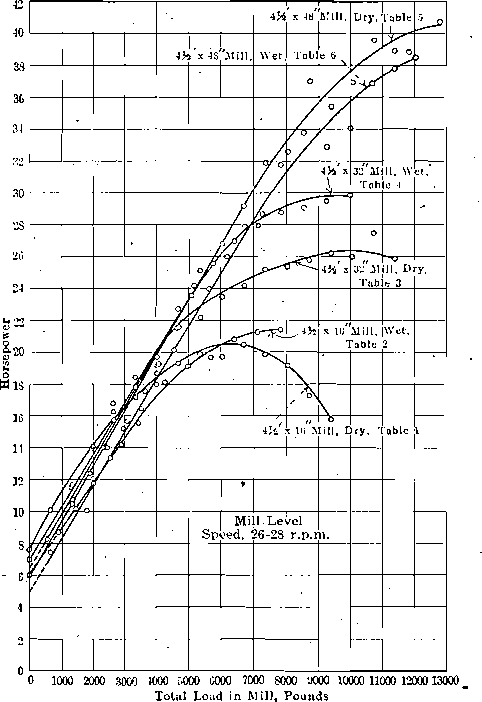Fig. 2- Power Consumption of 4 ½ -ft Conical Ball Mill at Hammond Laboratory

ula for horsepower. As will be seen by reference to this figure, the curves are closely parallel throughout their respective lengths, the only graph departing seriously from parallelism with, the others being that for the 4½ -ft. by 16-in. mill, dry, series No. 1. It will be noted that the varia­tion of this curve, begins at the point where the charge in the mill rose above the horizontal axis. The mill was here working under unnatural conditions, so that this variation will not affect a formula designed to cover working ranges only.

From this point two methods of procedure were followed, resulting in the following formulae for the horsepower of a conical ball mill within working ranges.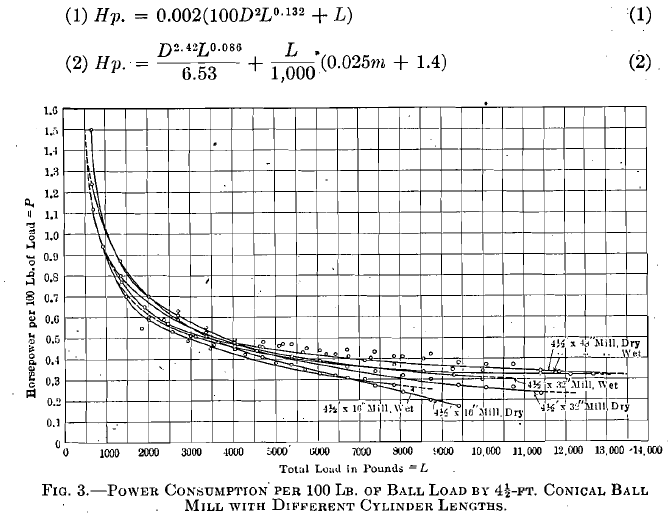where:

D = the internal diameter of the mill in feet,
L = the total load in the mill in pounds,
m = the length of the cylindrical section in inches.

The first of these formulae is of the nature of a preliminary trial and was developed from a free-hand average curve drawn through the curves on Fig. 3. It does not, therefore, give results which check throughout the range of operating conditions. Formula (2) was developed as outlined in the succeeding paragraph.

The first step in the determination of Formula (2) was to plot the average curve shown in Fig. 4 from the curves on Fig. 3. The points determining this curve were obtained by averaging the ordinates of the curves on Fig. 3 at the abscissae 1,000, 2,000, etc. Arbitrary ordinates y and abscissae x were then assigned to this curve and various functions such as x2 , y2 , x1/2 , y1/2 , log  x , log y , x/y , y/x , 1/x , 1/y, etc. were plotted against each other in different combinations man attempt to straighten out the curve. The best approximation to a straight line was obtained by plotting log {y — 2} as ordinates and log (x) as abscissae. The points thus obtained are shown on Fig. 5. The straight line drawn through these points was obtained by averaging ordinates and abscissae. The equation for this line is: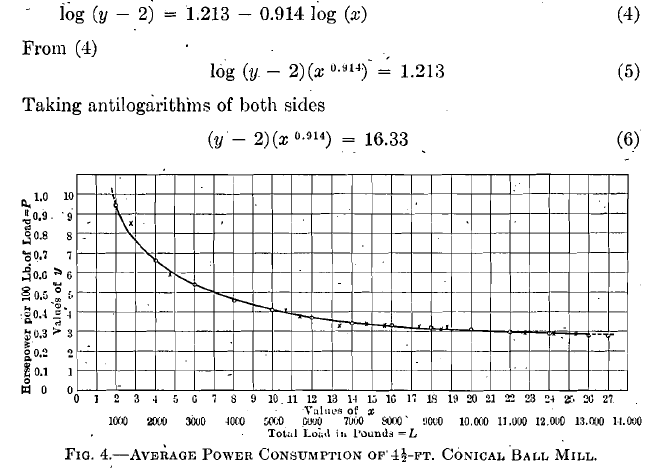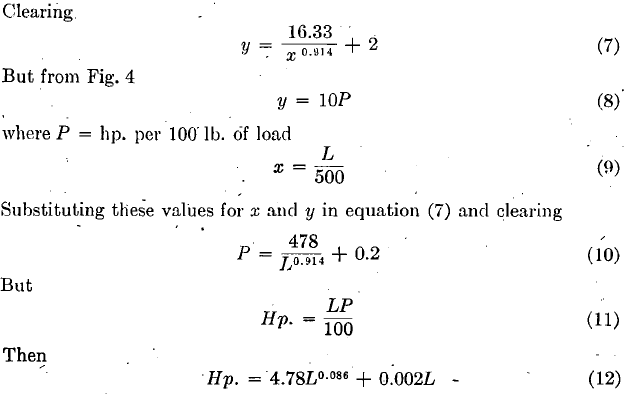Horsepowers solved for by this formula for different loads are indicated by crosses (x) on Fig. 4. These check solutions show a close agreement with the average curve, but show in some cases as much as 30 per cent, departure from the horsepowers determined experimentally. The variations are, as might be expected, greatest for the 16-in. and 48-in. cylindrical sections, as the average curve of Fig. 4 departs most greatly from the curves for these cylinder lengths. In order to eliminate this variation, Formula (12) was written as follows:

Hp. = 4.78 L0.086+ CL                                       (13)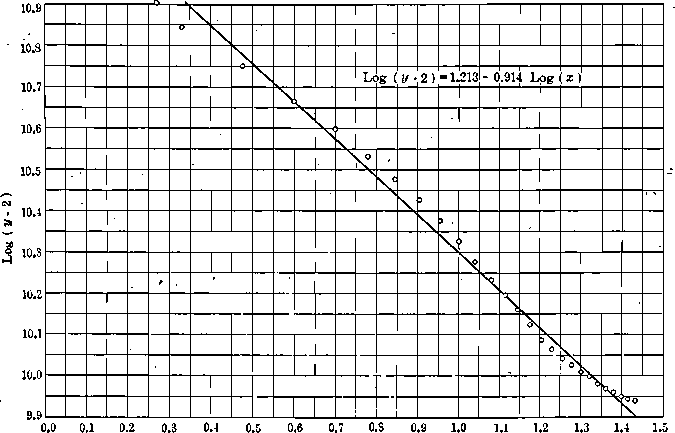Fig. 5- Log (x)

and average values of C were determined for different cylinder lengths by substituting known values of Hp. and L corresponding to values of m from results of tests, series 1 to 6 inclusive. By this method the follow­ing corresponding average values of C and m were determined:

 m C 16 0.0018 32 0.0022 48 0.0027

The relation between these quantities can be expressed in the linear form

C = 0.000025m + 0.0014                  (14)

from which equation (13) may be rewritten as

Hp   =    4.78L0.086 +L/1000 x (0.025m+1.4)               (15)

wluch gives values for the horsepower of the 4½-ft. ball mill that are accurate within a few per cent. The average curve for horsepower for mills of other diameters plotted with horsepower per 100 lb. (45.36 kg.) of load as ordinates and total load as abscissae will be similar to the curve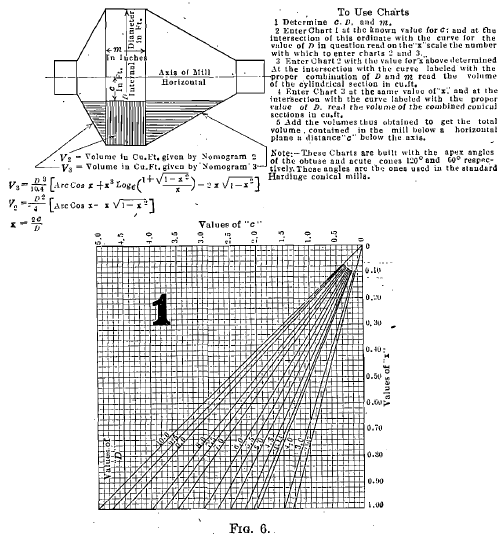in Fig. 4 and, by changing the scale, can be made to coincide with this curve. If, then, corresponding values of x and L on this figure can be established for mills of several diameters and the proper substitutions made in equation (7) we will get a series of different numbers for the coefficient of the term L0.086 in equation (15), corresponding to different internal diameters. The values of L corresponding to a given value of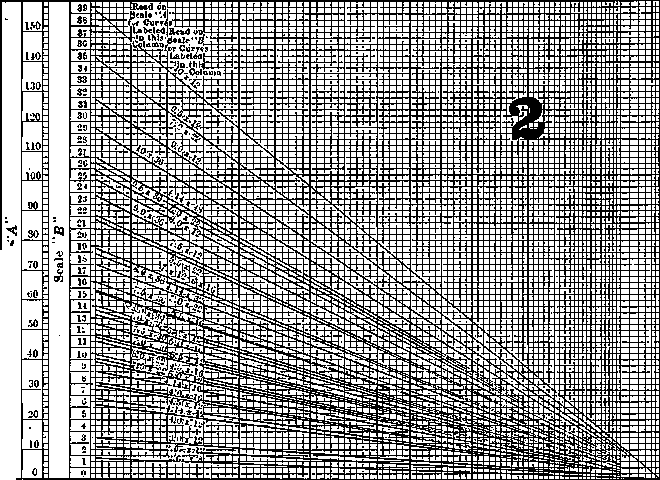Values of x from Nomogram 1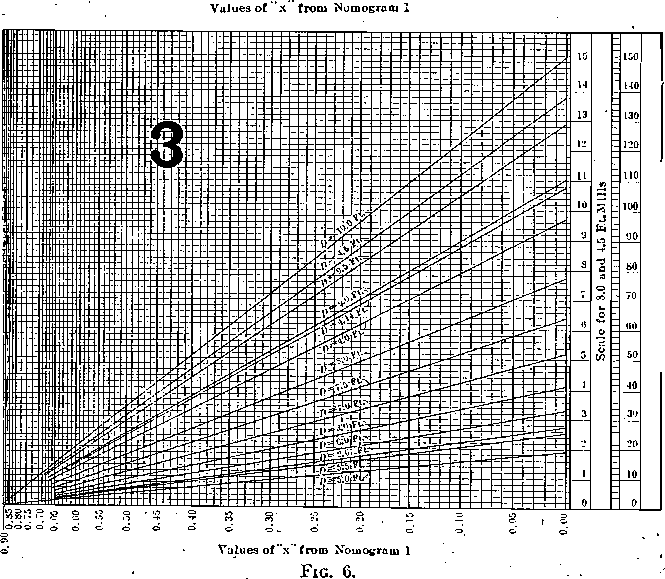x, Fig. 4, will vary according to the volumes of the different mills. These values and the corresponding values of the coefficients of L0.086 are as follows:

 Internal Diameter of Mill in feet x L Coefficient of L0.086 4.14 1 500 4.78 5.50 1 1,035 9.30 7.50 1 2,355 19.72 9.50 1 4,460 35.35

The logarithms of these coefficients of L0.086 and the logarithms of the internal diameter of the mill, D, bear a linear relation to each other which is expressed in the equation

log (D) = 0.413 log (C) + 0.337                            (16)

From this equation

C = D2.42/6.53 = coefficient of L0.086                    (17)

Substituting this value for the coefficient of L0 086 in equation (15) we have

Hp. = D2.42L0.086/6.53 + L/1000 X (0.025m + 1.4)                       (18)

This formula gives values accurate within a few per cent for. the horsepower of the conical ball mill throughout the range of operating conditions.

For pebble mills with smooth lining, results obtained by the above formula should be multiplied by the factor 0.65; with a semi-smooth lining, 0.8; with a rough lining, 0,95. It must be noted, however, in the use of the formula, that the load should be calculated on the assump­tion that the mill is horizontal, as the reduction in load due to tilting does not produce a corresponding decrease in power consumption.

The charts given in Figs. 6 and 7 will be found useful in determining the value of L in the horsepower formula. The use of these charts may be best explained by following through a calculation for the horsepower consumed by an 8-ft. by 30-in. ball mill crushing rock of a specific gravity of 2.6 with a moisture content of 50 per cent., using a 30,000-lb. ball load, composed of 5-in., 4-in., and 3-in. balls. For this condition D = 7.5.

c (Fig. 6) = 0. (The volume contained in a mill in operation is more than that contained in the same mill at rest, and the assumption that c = 0 is legitimate.)
Then from nomogram 1, x = 0.
Enter nomogram 3 with this value for x and read on line D = 7.5, V = 63.7.
Enter nomogram 2 with x = 0 aiid read on line 7.5X 30, V = 55.3.
The working volume of the mill is, then, 63.7 + 55.3 = 119.0 cu. ft.
The volume occupied by the balls is 30,000/495 = 60.6 cu. ft.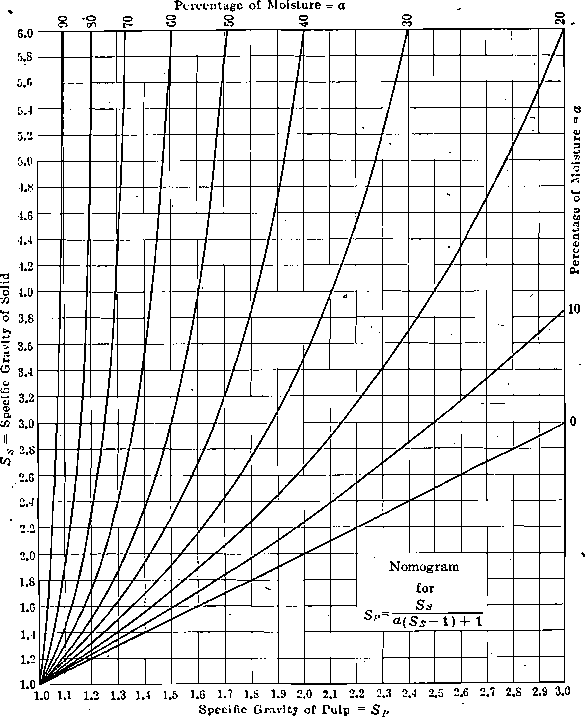Fig. 7

The volume occupied by the pulp is 119.0 — 60.6 = 58.4 cu. ft.
The specific gravity of the pulp is determined from Fig. 7.

Enter at Ss = 2.6. At the intersection with the curve a = 50 per cent., read SP = 1.44. The weight of the pulp in the mill is, then, 58.4(1.44)62.5 = 5,250 lb.

L = 30,000 + 5,250 = 35,250.

Hp. =  (7.52.42) (35,250)0.086/6.53 + 35,250/1,000 x (0.025(30)+1.4)
= 125.2

The economy in calculation to be gained from the use of Fig. 6 is not so apparent in the foregoing instance, where c was taken equal to zero, as it will be if the information sought is the crushing load which fills a given mill to within a given distance of the center, or the depth to which a given load will fill a mill of a given size. In the course of 2 or 3 years’ work with the mill the writer has been confronted with a consider-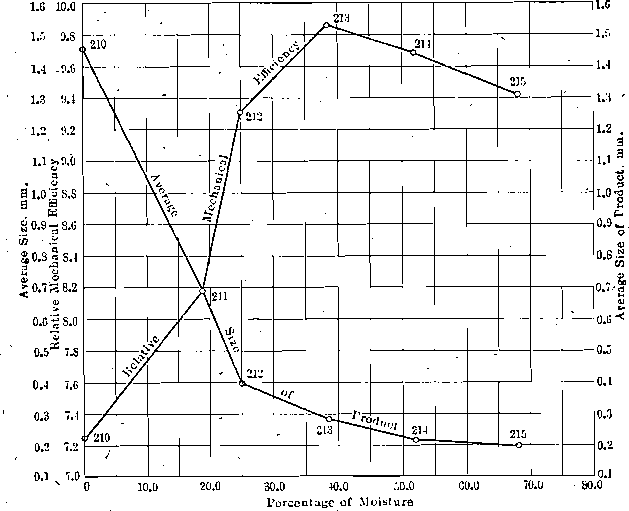Fig. 8- Effect of Moisture on Crushing Efficiency and Average Size of Product.

able number of such problems and it is because of the saving in time effected in their solution by the use of the chart, that it is inserted here. In such calculations the weight of a cubic foot of steel balls may be taken as 250 lb. and the weight of a cubic foot of pebbles as 100 lb.

Analysis of Operating Data:

Thirty-five tests were run on the 4½-ft. mill to determine the effect of variations in operating conditions on the performance of the mill. As each test furnished some information that may be classified under several heads, it is not possible, without considerable repetition, to segregate them. They are, therefore, presented in Tables 1 and 2 in the order in which they were performed and will be referred to by number in the subsequent discussion.

The indicator commonly used in this paper for comparing the charac­ter of the work done by the mill under any given condition with that done under some other condition is the figure in the last column of Table 1 headed R.M.E. (Relative Mechanical Efficiency). A detailed explanation of the development of this conception is given in the article,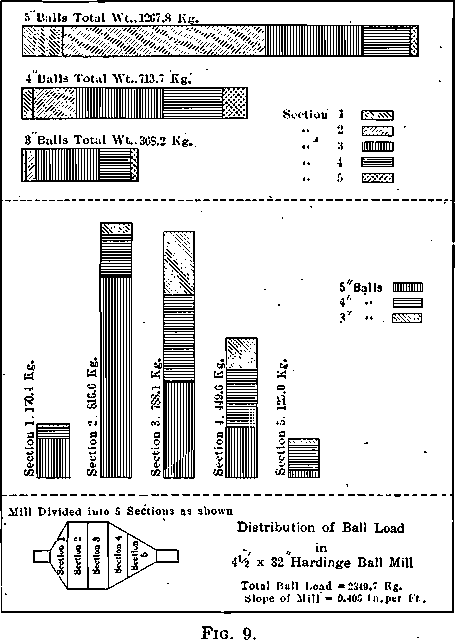The Work of Crushing, Trans. (1914), 48, 153. Briefly, it is expressed in the formula
R.M.E. = (Difference E.U. Feed and Product) (Tons per 24 hr.)/Hp.

in which the term “Difference E.U. Feed and Product” is a measure of the useful work done per unit of weight in reducing the material in ques­tion from feed size to discharge size, and is determined by screen analysis.

Thus the total energy units, E.U., in the feed sample, screen test 22-A, Table 2, is 34.32 and is obtained by summing the products of the percent­ages on the different screens by their corresponding ordinal numbers. The same method applied to screen test 22-41 gives 1,664.22 E.U. in the product of run No. 202. Then

R.M.E. = (1664.22 – 34.32) 12/21 = 930.

In order to make this figure accord with commonly accepted figures of efficiency, the R.M.E. thus obtained is divided by 100, giving for test 202 a value of 9.3.

Rate of Feed:

The effect of rate of feed on the relative mechanical efficiency of the conical-ball mill is given in the two groups of tests 202 to 204 and 213, 216 to 219. In the first group, trap rock of an average size of 24.58 mm. (0.96 in.) was fed dry at the rates of 1,000 lb. (453.59 kg.), 1,500 lb., and 2,000 lb. per hour. The axis of the mill was horizontal and the ball load was a mixture of 5-in., 4-in., 3-in., and 1 ¾-in. balls in approximately the same proportions that would-be found in commercial operation after the mill had settled down. The relative mechanical efficiencies, 9.3, 9.13 and 10.3 respectively indicate the result, confirmed in later tests, that the ratio of useful work done by the mill to power input increases ,with the feed rate. That there is, of course, a limit to this proportionate increase at the point of overload is shown in the second series of tests above mentioned. In this series the mill was tilted 2 5/8 in., or 0.405 in. per foot, toward the discharge end. One result of this tilting was to decrease the ball capacity of the mill by about 1,200 lb. Quartzite of an average size of 9.90 mm. was fed with an average of about 38 per cent, moisture at rates of 1,500 lb., 3,000 lb., 6,000 lb., 9,000 lb. and 12,000 lb. per hour. The relative mechanical efficiencies corresponding to the above rates were 9.86, 17.30, 29.21, 43.50, and 41.10. In this series of tests the relative mechanical efficiency of the machine increases with the feed rate up to 4.5 tons per hour, beyond which we have an apparent condition of overloading. Table 3 gives the reduction in average size of particle in the different tests above discussed.

These figures present three different cases for consideration. Test 202 is in a class by itself, the machine is patently underfed for all purposes except that of producing a practically finished, fine, dry product at one passage through the machine. It is a surprising fact that in doing this kind of work the machine uses power so efficiently. Tests 203, 204, 218 and 219 compared with tests 213, 216 and 217 point the moral that for most efficient work it is not wise to attempt too great reduction at one passage through the mill. When the large amount of power consumed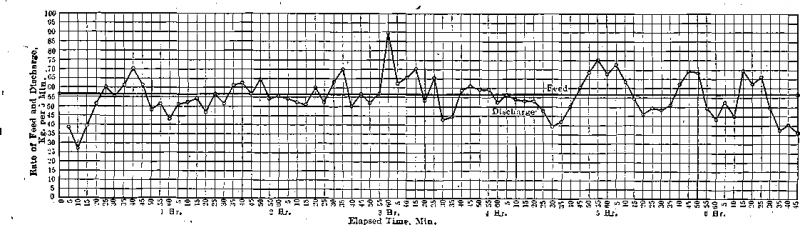Fig. 10- Comparison of Feed and Discharge Rates of Conical Ball Mill Dry Crushing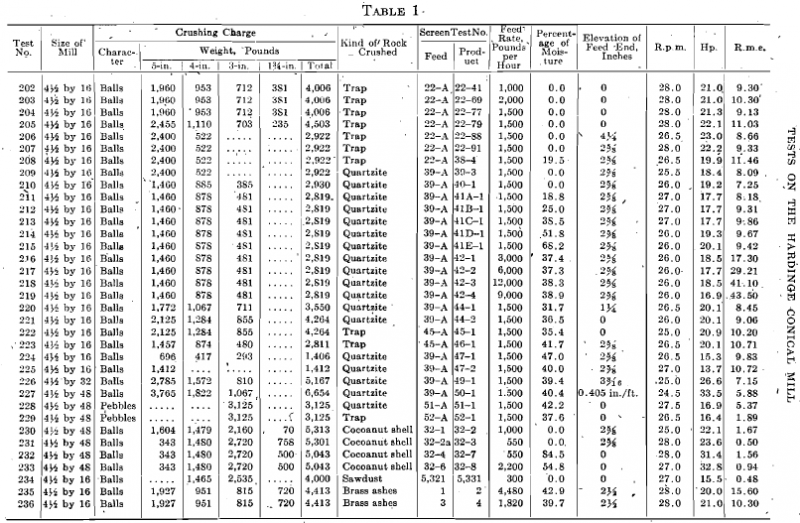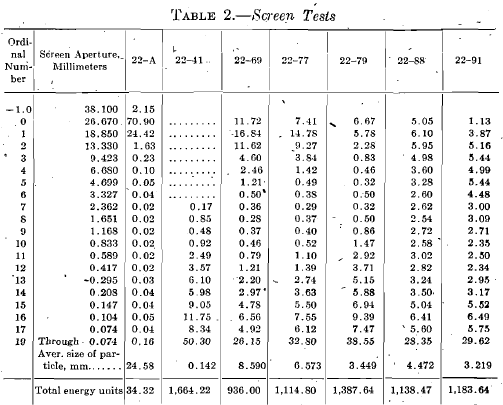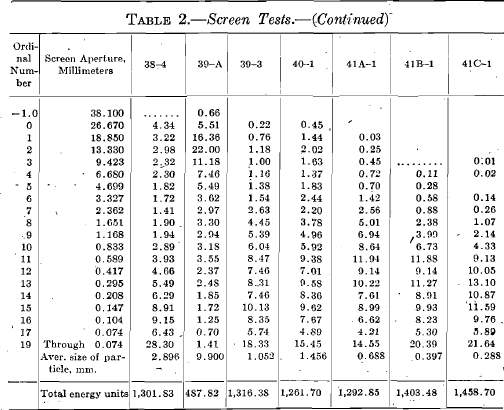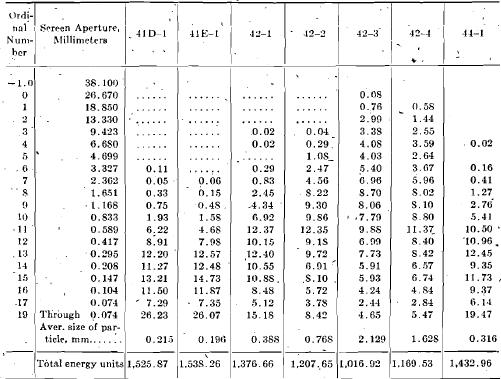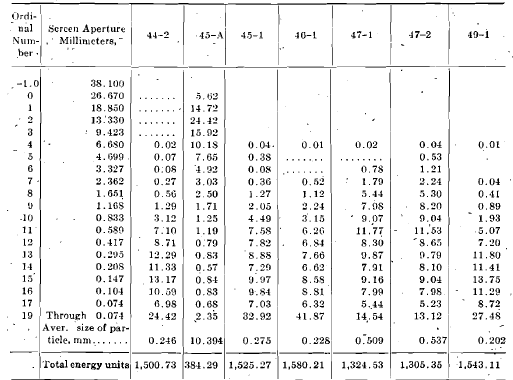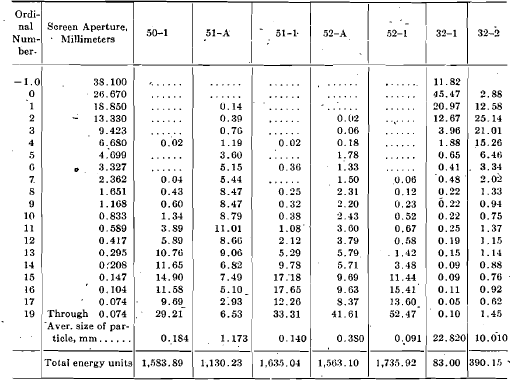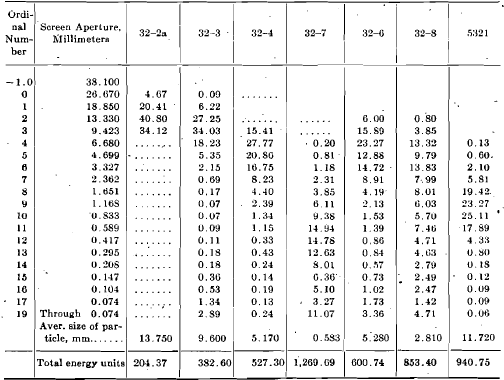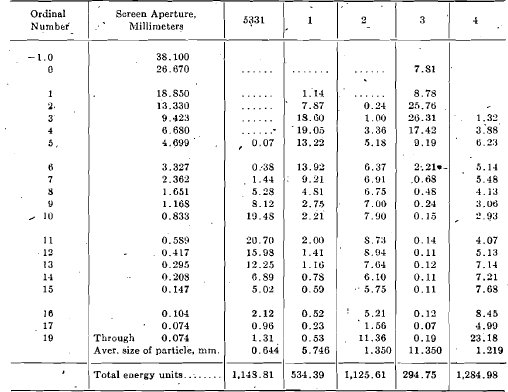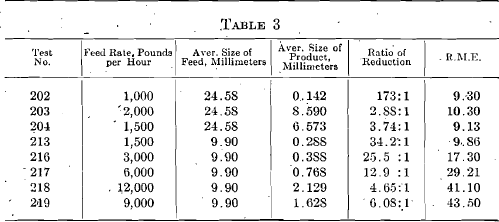by one of these mills is considered, together with the fact that the power consumption is practically the same whether the mill is loaded lightly or heavily, it should be apparent that it will pay well to expend the small amount of power necessary for handling the pulp in a closed circuit and thereby gain increased efficiency of the mill.

This conclusion is practically in accord with that reached by the usual method of analysis. Taking 0.295 mm. (48-mesh) as a limiting size sought, Table 4 shows the relative number of mills and, therefore, the relative amounts of power necessary to crush quartzite, the screen test of which is shown in S. T. 39-A, to pass a 0.295-mm. screen at the rate of 12,000 lb. per hour. The same relative figures will, of course, hold for any multiple of this desired capacity.

Table 4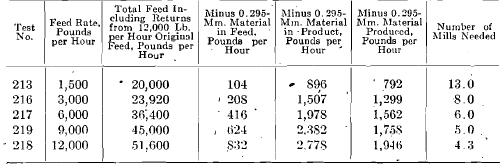This table is based on the assumption that the efficiency of reduction is the same on the smaller material returned to the mill as it is on the larger original feed. This is not quite true, but it is nearly enough true for the purposes of this argument.

Fig. 10 presents a fact which goes far toward, explaining the irregular performance often met with in machines following a crusher of the ball or  tube-mill type. It will be noted that at the end of the 6 ¾ hr. operation the divergence between feed and discharge rate at any given minute is as great as at the beginning of the run, despite a careful, regular feed. The rising portions of the curve are accompanied by a progressively coarser product. At the peaks the screen tests show but little crushing. This irregularity in discharge rate and character of product is greater in dry crushing than in wet crushing, but it is also distinctly apparent in wet crushing. In most mill practice the irregularity is smoothed out by crushing in closed circuit, the circuit acting as a balance. Where no such balance occurs through other features of mill design, it will be wise to make special provision if the machines treating the discharge

Effect of Moisture Content:

Fig. 8, summarizing tests 210 to 215 inclusive, Table 1, shows distinctly the effect of moisture on the crushing efficiency and average size of product of the conical ball mill. The true maximum of the efficiency curve probably lies somewhere between 40 and 50 per cent, moisture. The decidedly higher efficiency of wet crushing over dry crushing is confirmed in tests 207 and 208 where the relative mechanical efficiency rises from 9.33 to 11.46 due to the addition of 19 per cent, water, which is decidedly less than the most efficient water quantity. It will be noted, however, on referring to screen tests 40-1, 41A-1, 41B-1, 41C-1, 41D-1, and 41E-1, Table 2, that a progressively finer product is obtained by increasing the amount of water in the feed and that the decreased relative mechanical efficiency in tests 214 and 215 is due to increased power consumption.

Moisture content has an effect on the weight of crushing charge that can be held in a mill. If the mill is charged to the limit when pulp of a given-moisture content is being fed, a slight decrease in the moisture content will cause the discharge of a considerable quantity of balls or pebbles, as the case may be. The converse of this statement is of course, also true.

Effect of Slope:

The principal factors in mill operation affected by changes in slope are the ball load and the character of the product.

The effect on the ball load is best shown in tests 205 and 206. At the end of test 205 the mill, then level, contained-a charge of 4,503 lb. consisting of 2,455 lb. of 5-in., 1,110 lb. of 4-in., 703 lb. of 3-in. and 235 lb. of 1 ¾ -in. balls. At the end of test 206, which started with this load and was continued for several hours, the mill being set at a slope of 0.64-in. per foot, there had been forced out of the mill 55 lb. of 5-in., 588 lb. of 4-in., and all the 3-in. and 1 ¾-in. balls, leaving a total charge of 5-in. and 4-in. balls weighing but 2,922 lb. The ratio of weight of rock in the mill to Weight of balls was also reduced. This latter fact considerably lessens cushioning and increases the amount of crushing done by impact as compared to that done by abrasion. The result is reflected in the increased efficiency, and more granular product obtained, as noted later.

The change in power required to operate at higher slopes is in no way commensurate with what would be expected from the decrease in ball load (see tests 205 and 206). This fact should be borne in mind in using the formula given for horsepower.

The effect of changes in slope on the relative mechanical efficiency is so small that contradictory results due, no doubt, to unavoidable experimental inaccuracies, are shown. Thus tests 212, 213, 220 and 221 show a point of least efficiency at 1 ¼ -in. slope with higher efficiencies at 2 5/8-in. slope and no slope. It is the writer’s opinion that the relative mechanical efficiency increases with increase in slope within operating limits,, but that the change will in all cases be small. Tests 222 and 223 compared show higher efficiency and finer grinding at the greater slope. The finer grinding in test 223 is due to the higher moisture-content of the pulp, rather than to the increase in slope. The progressively coarser grinding with increasing slope in the quartzite series) tests 212, 213, 220 .and 221, is typical of the results to be expected in this direction. In dry grinding (see tests 205, 206, and 207) a decided change in the character of the product takes place with change of slope. The material discharged from the mill when grinding with the axis horizontal, contains a large percentage of — 200-mesh material and a considerable percentage of the coarsest sizes with a decided minimum in the amount of the intermediate sizes. The product, of the tilted mill, on the other hand, is more uniform. There is decidedly less coarse material and decidedly less dust, the bulk of the product lying in the intermediate sizes. This difference is undoubtedly due-to the difference in the character of the crushing done in the two cases. With the mill horizontal a considerable proportion of the load is rock. This rock acts as a cushion to the falling balls in the mill so that crushing by impact is greatly lessened and crush­ing by abrasion, forms an important part of the work done. In such crushing many of the large particles in the feed are reduced in size but slightly and pass out practically untouched, while such work as is effec­tive produces very fine material. Thus we have the large percentages of very coarse and very fine ingredients in the product. On the other hand, when the mill is tilted the amount of rock that it contains at any time is small in proportion to the crushing load, there is little or no cushioning and the amount of crushing done by impact is large in com­parison with that done by abrasion. Under such circumstances a granu­lar product is to be expected.

Effect of Difference in Size of Balls.—A comparison of test 209 with 210-and of test 224 with 225 shows that the larger the average size of ball in the crushing load (up to 5-in.- diameter) the smaller the power consumption and the higher the relative mechanical efficiency. It is to be further noted, that a mixture of 5-in. and 4-in. balls crushes finer than a mixture of 5-in., 4-in., and 3-in. balls of equal weight, when the crushing is done dry and the average size of the feed particles is 9.900 mm. (0.39 in.). When the work is done in the presence of water, as in tests 224 and 225, the product when the ball charge is a mixture of 5-in., 4-in., and 3-in. balls is slightly finer (0.509 mm. as against 0.539 mm.) than when the charge consists wholly of 5-in. balls, but in test 224 the moisture percentage was 47.0 per cent, as compared with 40.0 per cent, in test 225. By reference to the section on Effect of Moisture, it will be seen that this result is probably due to the difference in moisture content and that at the same moisture content the 5-in. balls would crush finer than the mixed charge. In any case the difference in fineness in favor of the mixed load is, so slight as to fail to justify charging a ball mill working on coarse feed with anything smaller than 5-in. balls. The writer inclines to the belief that the presence of small balls is a hindrance, and that periodical sorting of the charge accompanied by removal of the small balls (less than 3-in. diameter) will increase capacity, decrease power consumption, decrease the average size of the product and materially increase the relative mechanical efficiency.

Size of. Feed.—Comparison between tests 208 and 223 apparently indicates that the ball mill works more efficiently on a coarse feed (24.58 mm. (0.96 in.), average size) than on a finer feed (10.394 mm. (0.41 in.) average size). In test 223 the percentage of moisture present, 41.7 per cent., is practically that determined most favorable, while in 208, but 19.5 per cent, of water was present in the feed. Notwithstanding this fact the relative mechanical efficiency in crushing the larger feed is 11.46 as against 10.71 in the case of the finer feed. There is very little difference in the power consumption. This conclusion must, however, be limited by a statement as to the rate of feed, viz., 1,500 lb. per hour. The reduction ratio is but 7.02 in the ease of the larger feed as against, 45.6 for the finer feed. In neither case was the mill fed up to its most efficient capacity. Comparing the results obtained here with those obtained in the rate of feed tests (213 and 216 to 219 inclusive) we may expect that by pushing the capacity in the case of the smaller feed until the reduction ratio is in the neighbourhood of 7.0.that the relative mechan­ical efficiency will rise to about 40, while from the same series of tests it is obvious that lessening the ratio beyond this point in the case of the coarser feed, by increasing the feed rate, would result in lowering the relative mechanical efficiency. When these facts are taken into consideration the smaller feed gives most efficient operation.

This conclusion cannot, however, be extended to finer and finer feeds, as is apparent when the pebble mill runs on trap and quartzite, 228 and 229, are compared with the ball mill runs on the same rocks, tests 213 and 223. In the latter tests, with feeds of approximately the same average size, the efficiencies varied by but 7.9 per cent., the trap showing the higher result. In the pebble mill tests the average size of the quartz­ite feed was 1.173 mm. and of the trap feed 0.380 mm. The corre­sponding relative mechanical efficiencies were 5.37 and 1.89, a difference of 65 per cent., all of which must be ascribed to the fineness of the feed.

Length of Cylindrical Section.—The effect of increasing the length of the cylindrical section in a ball mill is to reduce the relative mechanical efficiency. This is due to the fact that the ball load and power consump­tion increase with increased length much more rapidly than the fineness of the product increases. Thus, by reference to Table 1 we find that, all conditions being constant other than those1 noted above, an increase in power consumption amounting to 89 per cent, occurs with an increase in length of cylindrical section from 16 in. to 48 in., the corresponding decrease in average size of product is but 36 per cent., and there is a resulting decrease in relative mechanical efficiency of 40 per cent., the 16-in. mill being taken as the standard of comparison. Therefore, if  the desired capacity of a plant is sufficient to justify the installation of more than one mill, additional mills placed in series, each making a relatively small reduction, will be more efficient than an installation ‘ which attempts a large reduction ratio in one mill by increasing the length of the cylindrical section.

Pebbles VS Balls:

Test 228 presents the pebble mill working at a reduction ratio of 8.3, which is close to the most economical ratio. Under these conditions the relative mechanical efficiency is 5.37. Test 227 presents a ball mill of the same cylinder length working on a coarser feed but making a reduction of 53.6 to 1. Even under this unfavourable condition the relative mechanical efficiency is 5.88. If the rate of feed is raised and the reduction ratio correspondingly lowered to a point comparable with the pebble mill, we may expect a relative mechanical efficiency much higher. It is ob­vious, then, that the ball mill is a more efficient crushing machine than the pebble mill. It is also obvious that it will grind as fine as the pebble mill, when the products of the two tests above cited are compared. The ball-mill product is 0.184 mm. average size, produced from a 9.9-mm. feed, while the pebble-mill product is 0.140 mm. average size produced from a feed only 1.173 mm. average size. Given a feed of the same size the ball-mill product would have been finer. Records were kept throughout of ball and pebble consumption, but  the results were so contradictory, due to the relatively short duration of the runs, that they are not worth presenting.

Character of   Feed:

When the feed to a ball mill is a rock similar to an average ore, no  great difference in efficiency is noticeable as between different kinds. Tests 221 and 222 give a comparison of grinding efficiencies on quartzite and trap of approximately the same average size. The reduction ratios in the two cases are 40.2 and 37.8 respectively, giving products 0.246 and 0.275 mm. average size. The relative mechanical efficiencies are 9.06 in the case of the quartzite-feed and 10.20 in the case of the trap-rock feed. When, however, tough, soft materials such as coconut shells or sawdust are tested (tests 230 to 234 inclusive) the efficiencies fall off rapidly to figures ranging from 0.48 to 1.67. This means, of course, that a crushing device employing impact chiefly is not suitable for, reducing such material.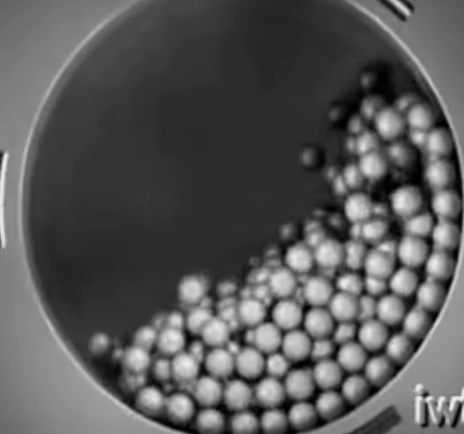An interesting and unexpected result is to be noted in tests 235 and 236. The brass ashes treated in these tests consisted of a mixture of unburned coal, coal ash, and a brittle slag containing metal shot. The coal ash and slag ground up with surprising ease, the coal was easily broken to an intermediate size and then seemed to float through on the surface  of the load in the mill, while the metal particles were discharged with  very little flattening or abrasion. Thus, due to the heterogeneous character of the feed, a higher efficiency was obtained than would be expected where one of the ingredients was so tough. When, however, it was attempted to grind slowly and pulverize metal, the relative mechanical efficiency of the machine fell to figures ranging from 0.04 to 0.71, confirming the comparison made in the first part of this section between rock and such tough materials as sawdust and coconut shells.

Capacity:

As stated in the introductory part of this paper, it has not been pos­sible to extend the series of tests to gain capacity, figures, on mills of different diameters. The writer has, however, some-figures on the capacity of 6-ft. and 8-ft. ball mills which indicate that with a feed of average ore of 10 mm. average diameter, grinding wet to pass a 20-mesh (0.833-mm.) screen, the capacity will vary as a function of the cube of the nominal diameter of the mill. Approximate capacities for this duty for mills 4.5 ft. diameter and larger may be derived from the formula

C = 0.95 D2 – 65

where C = the capacity in tons per 24 hr. and D = the nominal diameter of the mill in feet. This formula should, however, be used with caution.

Distribution of Crushing Charge:

At the end of one of the runs on the 4½ by 32 mill, the balls were sorted as they were taken from the mill into heaps corresponding to the portion of the mill from which they were removed. For the purpose of this classification the charge was divided by theoretical vertical planes into five sections, as shown in the diagrammatic sketch, Fig. 9. The heaps taken from each of these sections were then sorted into sizes, with the result shown graphically in Fig. 9. It will be seen from this figure that there is a marked segregation of large balls in sections 1 and 2 at the head end of the mill. The segregation is, however, by no means complete, as is shown by the fact that the average size of ball in the mixture in sections 3, 4, and 5 is greater than 4 in.

Conclusions:

1. In crushing average ores the character of the gangue has but little effect on the relative mechanical efficiency of the conical mill.
2. The mill is not suitable for grinding soft, tough materials.
3. The ball mill works more efficiently on material of intermediate. (0.5 in. to 0.75 in. average) size than on either a coarser or a finer feed.
4. A greater ratio of reduction in average size of material can be expected with feed of an intermediate size than with a coarse feed.
5. Steel balls are much more efficient crushing media than pebbles.
6. Steel balls will grind as fine or finer than pebbles when working on the same feed.
7. Increase in the weight of the ball load, other conditions remaining  constant, increases the ratio of reduction and the relative mechanical efficiency of the mill.
8. The power consumption increases with increase in the weight of the ball load, but this increase in power consumption is not in direct propor­tion to the increase in load.
9. Power consumption decreases with increase in the average size of the balls composing the crushing load up to an average size of 5 in.
10. A ball charge composed of 5-in. balls makes a greater reduction in size of particle at one passage through the mill than a mixed charge com­posed of 5-in., 4-in.; and 3-in. balls.
11. The relative mechanical efficiency of the ball mill increases with the average size of ball in the crushing charge up to 5 in. average diameter.
12. The relative mechanical efficiency of the mill increases with the  rate of feed to the point of overload.
13. Increase in the length of cylindrical section in the conical ball mill increases the reduction ratio at the expense of a marked decrease in the relative mechanical efficiency.
14. Increase in the slope of the mill axis decreases the ball load materially, but the corresponding decrease in power consumption is in no way commensurate.
15. In general, increase in slope tends to produce a more granular product with less very fine and less coarse ingredients than are present in the product of the mill set with the axis horizontal.
16. Increase in slope has but little effect on the relative mechanical efficiency.
17. Other conditions being constant, the relative mechanical efficiency of the mill is a maximum at between 40 and 50 per cent, moisture con­tent in the feed.
18. The relative mechanical efficiency in wet crushing is decidedly greater than in dry crushing.
19. The increase in the percentage of moisture in the feed causes, an increase in the reduction ratio.
20. Power consumption increases slightly with increase in the moisture content of the feed.
21. The rate of discharge and the character of the product of the mill fluctuate continually through rather wide limits. This fluctuation is greatest in dry crushing.
22. The conical mill should be operated in closed circuit with a sizing device which will return to it the oversize from its product. In this installation the rate of feed should be raised until the relative mechanical efficiency shows a maximum. When operating as a ball mill, the ratio of length of cylindrical section to diameter should not exceed 0.3. This will be a much more economical installation than one which seeks, by slow feeding or long cylindrical section, to obtain a finished product at one passage through the mill. In wet grinding the moisture content of the feed should be kept about 40 per cent. The slope-should be adjusted to mill requirements, but for ordinary concentrating-mill practice should be about 0.4.in. per foot. The ball charge should be the maximum that the mill will hold and should be kept as large in average size as is possible without too great sacrifice of small balls.